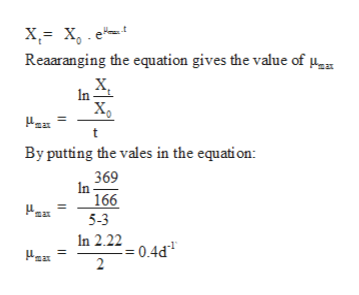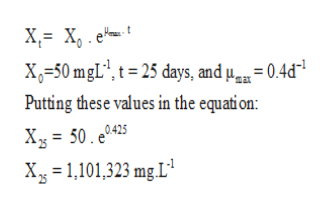# (Chart Given) Time (d)    Biomass(mg/L)0                      501                      752                     1113                    1664                     2485                     369a. Calculate u(max).b. What would the population size be after 25 days? (answer a and b)

Question

(Chart Given)
Time (d)    Biomass(mg/L)
0                      50
1                      75
2                     111
3                    166
4                     248
5                     369

a. Calculate u(max).

b. What would the population size be after 25 days?

check_circleExpert Solution
Step 1

To calculate µmax the for the population and size of population after 25 days.

Step 2

Using exponential growth equation, the value of µmax can be calculated:help_outlineImage TranscriptioncloseReaaranging the equation gives the value of n Xg By putting the vales in the equati on: 369 In 166 max 5-3 In 2.2204d 2 fullscreen
Step 3

Population size can be cal...help_outlineImage TranscriptioncloseX X, e X=50 mgL', t=25 days, and u= 0.4d* Putting these values in the equation: Xy= 50. e0425 Xg 1,101,323 mg L fullscreen

### Want to see the full answer?

See Solution

#### Want to see this answer and more?

Solutions are written by subject experts who are available 24/7. Questions are typically answered within 1 hour*

See Solution
*Response times may vary by subject and question
Tagged in

### Biology